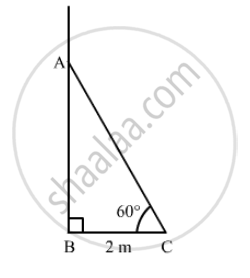Advertisement Remove all ads

# A Ladder Makes an Angle of 60º with the Ground When Placed Against a Wall. If the Foot of the Ladder is 2 M Away from the Wall, Then the Length of the Ladder (In Metres) is - Mathematics

MCQ

A ladder makes an angle of 60º with the ground when placed against a wall. If the foot of the ladder is 2 m away from the wall, then the length of the ladder (in metres) is

#### Options

• $\frac{4}{\sqrt{3}}$

• $4\sqrt{3}$

• $2\sqrt{2}$

• $4$

Advertisement Remove all ads

#### SolutionSuppose AC is the ladder and BC is the distance of the foot of the ladder from the wall.
It is given that, BC = 2 m and $\angle$ACB = 60°.

In right ∆ABC,

$\cos60° = \frac{BC}{AC}$
$\Rightarrow \frac{1}{2} = \frac{2}{AC}$
$\Rightarrow AC = 4 m$

Is there an error in this question or solution?
Advertisement Remove all ads

#### APPEARS IN

RD Sharma Class 10 Maths
Chapter 12 Trigonometry
Q 27 | Page 44
Advertisement Remove all ads

#### Video TutorialsVIEW ALL 

Advertisement Remove all ads
Share
Notifications

View all notifications

Forgot password?
Course